# Assignment Solution of Conditional Statements

## Assignment Solution for Conditional Statements

In this article, I am going to give you the solutions that we give you as an assignment in our Conditional Statements and Logical Operations article. So, please read our Conditional Statements and Logical Operations if you have not read yet. First, try to solve the problem by yourself and then only look at the below solutions.

##### Program to divide two numbers validate a condition if the denominator is zero it should pop a message division by zero is impossible.

Note: This program is an example of usage of conditional if statement without else. The conditional if is usually used to perform validation operations like below.

Solution:

```#include <iostream>
using namespace std;
int main ()
{
int a, b, result, choice;
cout << "Enter the value of a and b : " << endl;
cin >> a >> b;
if (b == 0)
{
cout << "Division by zero is impossible" << endl;
return 0;
}
result = a / b;
cout << "Result is : " << result << endl;
return 0;
}
```

Output: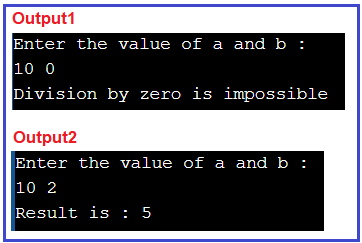##### Program to find the maximum of three numbers.

This program can be implemented using nested if and also using a ladder if.

###### Solution 1 using a ladder if:
```#include <iostream>
using namespace std;
int main ()
{
int a, b, c;
cout << "Enter the value of a b and c : " << endl;
cin >> a >> b >> c;
if (a > b && a > c)
{
cout << a;
}
else if (b > a && b > c)
{
cout << b;
}
else
{
cout << c;
}
return 0;
}
```

Output: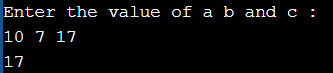###### Solution 2 using nested if:
```#include <iostream>
using namespace std;
int main ()
{
int a, b, c;
cout << "Enter the value of a b and c : " << endl;
cin >> a >> b >> c;
if (a > b)
{
if (a > c)
{
cout << a;
}
else
{
cout << c;
}
}
else if (b > a)
{
if (b > c)
{

cout << b;
}
else
{
cout << c;
}
}
return 0;
}
```

Output: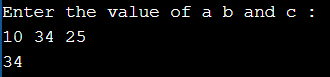##### Program to Check if the number is odd or even

Solution:

```#include <iostream>
using namespace std;
int main ()
{
int a;
cout << "Enter the value of a : " << endl;
cin >> a;
if (a % 2 == 0)
{
cout << "even";
}
else
{
cout << "odd";
}
return 0;
}
```

Output: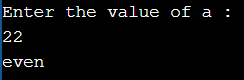##### Program to Check if the number is positive or not

Solution:

```#include <iostream>
using namespace std;
int main ()
{
int a;
cout << "Enter the value of a : ";
cin >> a;
if (a >= 0)
{
cout << "Positive";
}
else
{
cout << "Negative";
}
return 0;
}
```

Output: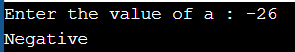##### Program to display the month name. For example, if the user input 11 it should show November.

This is an example program for the ladder if and switch case as well.

```#include <iostream>
using namespace std;
int main ()
{
int a;
cout << "Enter which month name do you want, valid months are 1-12 : ";
cin >> a;
if (a == 1)
{
cout << "JANUARY";
}
else if (a == 2)
{
cout << "FEBRUARY";
}
else if (a == 3)
{
cout << "MARCH";
}
else if (a == 4)
{
cout << "April";
}
else if (a == 5)
{
cout << "MAY";
}
else if (a == 6)
{
cout << "JUNE";
}
else if (a == 7)
{
cout << "JULY";
}
else if (a == 8)
{
cout << "AUGUST";
}
else if (a == 9)
{
cout << "SEPTEMBER";
}
else if (a == 10)
{
cout << "OCTOBER";
}
else if (a == 11)
{
cout << "NOVEMBER";
}
else if (a == 12)
{
cout << "DECEMBER";
}
else
{
cout << "Invalid Month";
}
}
```

Output: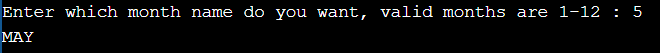###### Solution 2:
```#include <iostream>
using namespace std;
int main ()
{
int a;
cout << "Enter which month name do you want, valid months are 1-12 : ";
cin >> a;
switch (a)
{
case 1:
cout << "JANUARY";
break;
case 2:
cout << "FEB";
break;
case 3:
cout << "MARCH";
break;
case 4:
cout << "APRIL";
break;
case 5:
cout << "MAY";
break;
case 6:
cout << "JUN";
break;
case 7:
cout << "JUL";
break;
case 8:
cout << "AUG";
break;
case 9:
cout << "SEP";
break;
case 10:
cout << "OCT";
break;
case 11:
cout << "NOV";
break;
case 12:
cout << "DEC";
break;
default:
cout << "Invalid month number";
}
return 0;
}```

Output: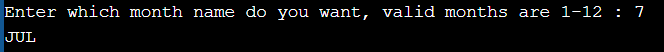##### Program to check whether the alphabet is vowel or consonants using switch statements.
```#include <iostream>
using namespace std;
int main ()
{
char a;
cout << "Enter Alpbhaet name A-Z : ";
cin >> a;
if ((a >= 'A' && a <= 'Z') || (a >= 'a' && a <= 'z'))
{
switch (a)
{
case 'A':
case 'E':
case 'I':
case 'O':
case 'U':
case 'a':
case 'e':
case 'i':
case 'o':
case 'u':
cout << "Vowel";
break;
default:
cout << "Consonant";
}
}
else
{
cout << "Enter a valid alphabet" << endl;
}
return 0;
}
```

Output: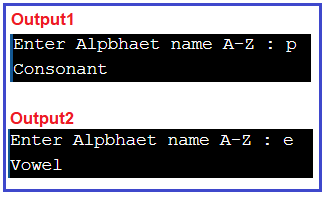That’s it for today. We have given the solutions that we give you as an assignment in our Conditional Statements and Logical Operations article. If you have a better solution, then please post your solution in the comment box so that other guys will get benefits.